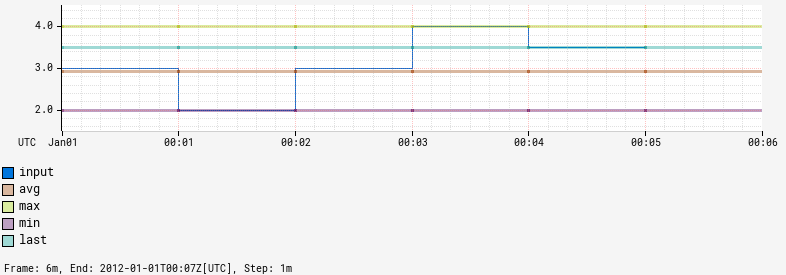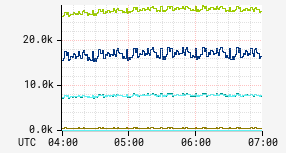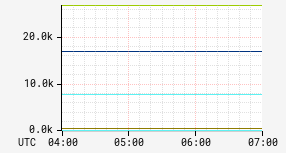# stat

Input Stack:
 String TimeSeriesExpr
Output Stack:
 TimeSeriesExpr

Create a summary time series showing the value of the specified summary statistic for the data points of the input time series. Valid statistic values are `avg`, `count`, `max`, `min`, `last`, and `total`. The graph below shows `avg`, `max`, `min`, and `last` for a simple input time series:The `count` is the number of data points for the time series. In the example above, that is five since the last value is `NaN`. The `total` is the sum of the data points for the time series.

The most common usage of stats is in conjunction with :filter to restrict the set of results for grouped expression. When filtering, helper macros, `:stat-\$(name)`, can be used to represent applying the statistic to the input time series being filtered without explicitly repeating the input expression.

Example of usage:

 Before After```name,sps,:eq, (,nf.cluster,),:by ``` ```name,sps,:eq, (,nf.cluster,),:by, avg,:stat ```